# Java Variables

A variable is the fundamental unit of storage in a Java program. An identifier, a type, and an optional initializer are used to define a variable. Furthermore, each variable has a scope that defines its visibility as well as a lifetime. A separate (next) post will teach you about the scope of variables.

## Java Variable Declaration

In Java, all the variables must be declared before they can be used. The basic form of a variable declaration in Java is shown here:

`type identifier;`

or,

`type identifier = value;`

or,

`type identifier1, identifier2, ... ;`

Here, "type" is one of Java's types or the name of a class or interface. The "identifier" is the name of the variable. You can initialize the variable by specifying an equal sign and a value.

The identifier is the name of variable. You can initialize the variable by specifying an equal sign and a value.

Note: Keep in mind that the initialization expression must result in a value of the same or compatible type as that specified for the variable.

To declare more than one variable of the specified type, use a comma-separated list.

Following are some examples of variable declarations of various types. Note that some include an initialization.

```int a, b, c;
int d = 3, e, f = 5;
byte z = 22;
char x = 'x';```

The names of the identifiers you select reveal nothing about their types. Any properly formed identifier in Java can have any declared type.

## Java Variable Types

A variable is essentially the memory location's name. Memory layout and size can differ depending on the data type of a variable. Following is a list of all three types of variables available in Java.

### Local variables in Java

Local variables in Java are created locally, such as within a method, constructor, or any block, and are automatically destroyed when the method, constructor, or block where they were created is exited. Here is an example program that demonstrates the concept and use of local variables in Java. Here marks the local variable.

```public class JavaProgram
{
void printMarks()
{
int marks = 0;
marks = marks + 96;
System.out.println("Marks of Deepak = " + marks);
}
public static void main(String[] args)
{
JavaProgram jp = new JavaProgram();
jp.printMarks();
}
}```

Here is a sample of the output that the aforementioned Java program produced: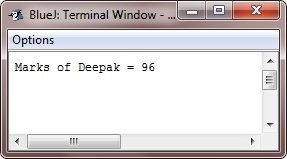### Instance variables in Java

When the "new" operator is used to make an object in Java, instance variables are made.In Java, instance variables can be created in a class but not in any block, method, or constructor. Here is an example program that illustrates the concept and use of instance variables in Java:

```import java.io.*;

public class JavaProgram
{
public String studname;      // This instance variable is visible for any child class.
private String studbranch;   // This instance variable is only visible to the JavaProgram class.

public JavaProgram(String name)
{
studname = name;
}
public void initializeBranch(String branch)
{
studbranch = branch;
}
public void printStudent()
{
System.out.println("Name = " + studname);
System.out.println("Branch = " + studbranch + "\n");
}

public static void main(String args[])
{
JavaProgram jp1 = new JavaProgram("Deepak");
jp1.initializeBranch("CSE");
jp1.printStudent();
JavaProgram jp2 = new JavaProgram("Rajat");
jp2.initializeBranch("Electrical");
jp2.printStudent();
}
}```

Here is the sample output produced by this Java program: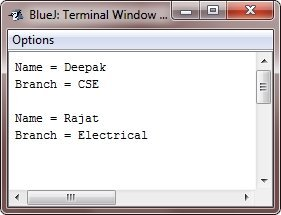### Static variables in Java

Using the "static" keyword outside of any block, method, or constructor in a class makes a static variable, also called a class variable. Here is an example program that helps you understand the static variables in Java:

```import java.io.*;

public class JavaProgram
{
private static String studname;
public static final String studbranch = "IT";

public static void main(String args[])
{
studname = "Deepak";
System.out.println(studname + " is in " + studbranch + " branch");
}
}```

The above Java program will produce the following output: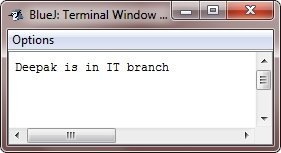## Java Type Conversion

Programmers know that assigning a value of one type to a variable of another type is common. Java automatically converts compatible types. "int" values can be assigned to "long" variables.

### Java's Automatic Conversion

If the following two requirements are satisfied, an automatic type conversion will occur when one type of data is assigned to another type of variable:

• The two types are compatible.
• The destination type is larger than the source type.

A widening conversion happens when these two conditions are satisfied. For instance, there is never a need for an explicit cast statement because the int type can hold all legitimate byte values.

The numeric types, including the integer and floating-point types, are compatible with one another for widening conversions. The conversion of numeric types to char or boolean is not automatic, though. Additionally, char and boolean are incompatible.

Java also automatically converts the type of a literal integer constant when storing it in byte, short, long, or char variables.

The following program demonstrates the type conversion in Java:

```class TypeConversionDemo
{
public static void main(String args[])
{

char ch = 'A';
int a, b = 68;
long x;

x = b;
System.out.println("x(long) = b(int with value 68) : " + x);

a = ch;
System.out.println("a(int) = ch(char with value A) : " + a);
}
}```

When the above Java program is compiled and executed, it will produce the following output: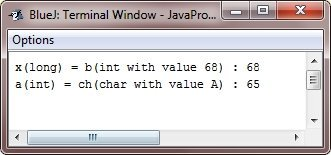### Java Automatic Type Promotion

Aside from assignments, there is another place where certain type conversions can occur: expressions. Consider the following. The precision required of an intermediate value in an expression will occasionally exceed the range of either operand. Consider the following expression:

```byte a = 40;
byte b = 50;
byte c = 100;
int d = a * b / c;```

The output of the intermediate term, a * b, easily exceeds the range of either of its "byte" operands.

To handle this type of problem, Java automatically promotes each byte, short, or char operand to an int when evaluating an expression. It means that the subexpression a*b is performed using integers, not bytes. Thus, 2,000, the result of the intermediate expression, 50 * 40, is legal even though a and b are both specified as being of type byte.

As useful as the automatic promotions are, they can cause confusing compile-time errors as well. For example, this seemingly correct code causes a problem:

```byte b = 50;
b = b * 2;     // Error! can't assign an int to a byte!```

The code is attempting to store 50*2, an absolutely valid byte value, back into a byte variable. However, because the operands were automatically promoted to an int when the expression was evaluated, the result has also been promoted to an int. Therefore, the result of the expression is now of type int, which cannot be assigned to a byte without the use of a cast. This is true even if, as in this case, the value being assigned would still fit in the target type.

In cases where you understand the effect of overflow, you should use an explicit cast, such as

```byte b = 50;
b = (byte) (b * 2);```

which yields the correct value of 100.

#### Java Type Promotion Rules

Java defines several type promotion rules that apply to the expressions.

They are as follows: First, all the byte, short, and char values are promoted to an int, as just described. Then, if one operand is a long, the whole expression is promoted to long. If one operand is a float, the entire expression is promoted to float. If any of the operands are double, the result is double.

The following program shows how each value in an expression is moved up to match the second argument to each binary operator.

```public class JavaProgram
{
public static void main(String args[])
{

byte b = 42;
char c = 'a';
short s = 1024;
int i = 50000;
float f = 5.67f;
double d = .1234;

double result = (f * b) + (i / c) - (d * s);

System.out.println((f * b) + " + " + (i / c) + " - " + (d * s));
System.out.println("result = " + result);
}
}```

When the above Java program is compiled and executed, it will produce the following output: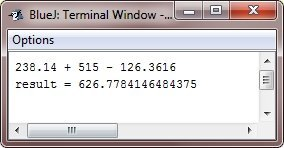Let's look closely at the types of promotions that occur in this line from the above program:

double result = (f * b) + (i / c) - (d * s);

The result of the first subexpression, (f * b), is float because "b" is promoted to a float. Following that, "c" is promoted to int in the subexpression (i / c), and the result is of type "int." The value of "s" is then promoted to double in (d * s), and the type of the subexpression is "double." Finally, these three intermediate values are considered: float, int, and double. A float is the result of a float plus an int. The resultant float minus the last double is then promoted to double, which is the type of the expression's final result.

Java Online Test

« Previous Tutorial Next Tutorial »